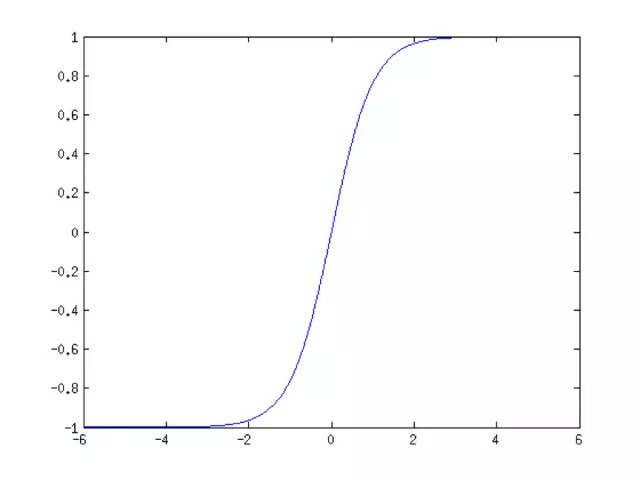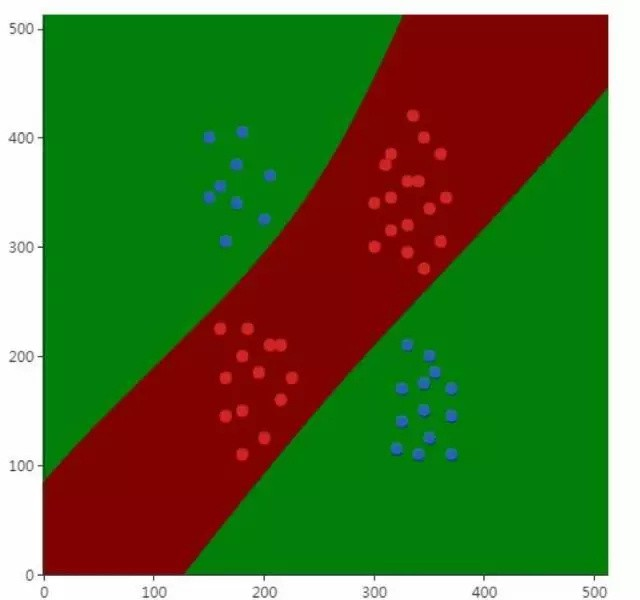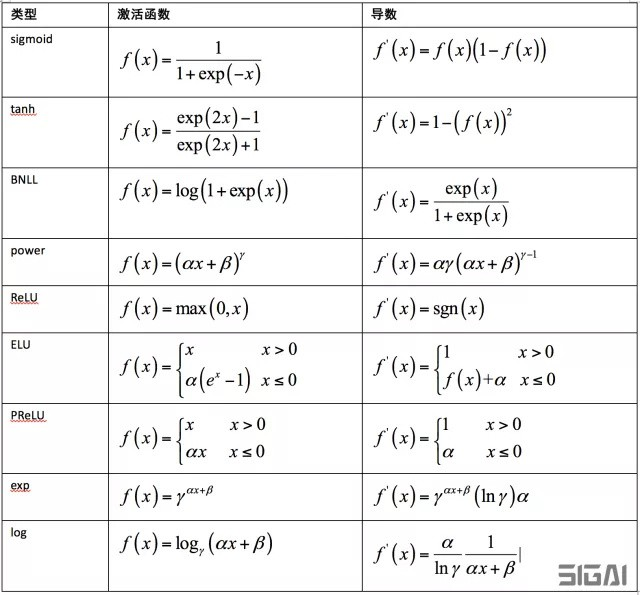## 1. 为什么需要激活函数

$$R^n \rightarrow R^m$$

$$y = h(x)$$

$$\begin{matrix} u^{(l)} = W^{(l)} x^{(l-1)} + b^{(l)} \\ x^{(l)} = f(u^{(l)}) \end{matrix}$$

$$h(x) = f(W^{(3)} f(W^{(2)} f(W^{(1)}x + b^{(1)}) + b^{(2)}) + b^{(3)})$$

$$W^{(3)} (W^{(2)} (W^{(1)}x + b^{(1)}) + b^{(2)}) + b^{(3)}$$

$$f(x) = \frac{1}{1 + exp(-x)}$$tanh函数的计算公式为：

$$f(x) = \frac{1 - e^{-2x}}{1 + e^{-2x}}$$## 2. 什么样的函数可以做激活函数

$$F(\mathbf{x}) = \sum_{i=1}^N v_i \varphi (\mathbf{w}_i^T \mathbf{x} + b_i)$$

$$|F(\mathbf{x}) - f(\mathbf{x})| < \epsilon$$

$$\begin{matrix} lim_{x \rightarrow -\infty} \sigma(x) = 0 \\ lim_{x \rightarrow +\infty} \sigma(x) = 1 \end{matrix}$$

$$f(x) = \sum \alpha_i \sigma(\mathbf{w}_i^T \mathbf{x} + b_i)$$

$$ReLU(x) = max(0, x)$$$$\delta^{(l)} = (W^{(l+1)})^T \delta^{(l+1)} \odot f'(u^{(l)})$$

## 3. 什么样的函数是好的激活函数

$$\frac{x}{1 + |x|}$$

$$W_{ij} = U[- \frac{1}{\sqrt{n}}, \frac{1}{\sqrt{n}}]$$

$$\lim\limits_{x \rightarrow + \infty} f'(x)=0$$

$$\lim\limits_{x \rightarrow -\infty} f'(x)=0$$

sigmoid函数的输出映射在(0,1)之间，单调连续，求导容易. 但是由于其软饱和性，容易产生梯度消失，导致训练出现问题；另外它的输出并不是以0为中心的.

tanh函数的输出值以0为中心，位于(-1,+1)区间，相比sigmoid函数训练时收敛速度更快，但它还是饱和函数，存在梯度消失问题.

ReLU函数其形状为一条折线，当x<0时做截断处理. 该函数在0点出不可导，如果忽略这一个点其导数为sgn. 函数的导数计算很简单，而且由于在正半轴导数为1，有效的缓解了梯度消失问题. 在ReLU的基础上又出现了各种新的激活函数，包括ELU、PReLU等. 如果对各种激活函数深入的比较和分析感兴趣，可以阅读文献.

## 4. 常用的激活函数## 5. Caffe 中激活函数的具体实现

### 5.1. SigmoidLayer

SigmoidLayer类实现了标准sigmoid激活函数. 正向传播函数对每个输入数据计算sigmoid函数值，在这里count是输入数据的维数. 实现代码如下：

sigmoid_layer.cpp

template <typename Dtype>
void SigmoidLayer<Dtype>::Forward_cpu(
const vector<Blob<Dtype>*>& bottom,
const vector<Blob<Dtype>*>& top) {
// 输入数据
const Dtype* bottom_data = bottom->cpu_data();
// 输出数据
Dtype* top_data = top->mutable_cpu_data();
// 输入数据的个数
const int count = bottom->count();
for (int i = 0; i < count; ++i) {
// 对每个数据计算 sigmoid 函数
top_data[i] = sigmoid(bottom_data[i]);
}
}

template <typename Dtype>
void SigmoidLayer<Dtype>::Backward_cpu(
const vector<Blob<Dtype>*>& top,
const vector<bool>& propagate_down,
const vector<Blob<Dtype>*>& bottom) {
if (propagate_down) {
// top_data 本层的 sigmoid 值
const Dtype* top_data = top->cpu_data();
// top_diff 为后一层的误差
const Dtype* top_diff = top->cpu_diff();
// bottom_diff 保存了本层的误差
Dtype* bottom_diff = bottom->mutable_cpu_diff();
const int count = bottom->count();
for (int i = 0; i < count; ++i) {
// sigmoid 函数值在正向传播时被计算出来，
// 并存在这里以供反向传播时使用.
const Dtype sigmoid_x = top_data[i];
// 计算 sigmoid 函数的导数，并与后一层传来的误差相乘.
bottom_diff[i] = top_diff[i] * sigmoid_x * (1. - sigmoid_x);
}
}
}

### 5.2. TanHLayer

TanHLayer类实现了tanh激活函数. 正向传播函数实现代码如下：

tanh_layer.cpp

template <typename Dtype>
void TanHLayer<Dtype>::Forward_cpu(
const vector<Blob<Dtype>*>& bottom,
const vector<Blob<Dtype>*>& top) {
const Dtype* bottom_data = bottom->cpu_data();
Dtype* top_data = top->mutable_cpu_data();
const int count = bottom->count();
// 对每个输入函数计算 tanh 函数
for (int i = 0; i < count; ++i) {
// 直接调用数学库的 tanh 函数
top_data[i] = tanh(bottom_data[i]);
}
}

template <typename Dtype>
void TanHLayer<Dtype>::Backward_cpu(
const vector<Blob<Dtype>*>& top,
const vector<bool>& propagate_down,
const vector<Blob<Dtype>*>& bottom) {
if (propagate_down) {
const Dtype* top_data = top->cpu_data();
const Dtype* top_diff = top->cpu_diff();
Dtype* bottom_diff = bottom->mutable_cpu_diff();
const int count = bottom->count();
Dtype tanhx;
for (int i = 0; i < count; ++i) {
tanhx = top_data[i]; // 取得 tanh(x) 的值
// 计算 tanh 的导数，并与后一层的误差相乘.
// 导数计算公式可见上一章节中的表格.
bottom_diff[i] = top_diff[i] * (1 - tanhx * tanhx);
}
}
}

### 5.3. ELULayer

ELULayer类实现ELU激活函数，是直线函数和指数函数的结合.

$$f'(x) = \alpha e^x = \alpha e^x - \alpha + \alpha = \alpha(e^x - 1) + \alpha = f(x) + \alpha$$

elu_layer.cpp

template <typename Dtype>
void ELULayer<Dtype>::Forward_cpu(
const vector<Blob<Dtype>*>& bottom,
const vector<Blob<Dtype>*>& top) {
const Dtype* bottom_data = bottom->cpu_data();
Dtype* top_data = top->mutable_cpu_data();
const int count = bottom->count();
Dtype alpha = this->layer_param_.elu_param().alpha();
for (int i = 0; i < count; ++i) {
// 当 bottom_data[i]>0 时，下时的值为 bottom_data[i]；
// 否则，值为 alpha * exp(bottom_data[i] - 1)
top_data[i] = std::max(bottom_data[i], Dtype(0))
+ alpha * (exp(std::min(bottom_data[i], Dtype(0))) - Dtype(1));
}
}

template <typename Dtype>
void ELULayer<Dtype>::Backward_cpu(
const vector<Blob<Dtype>*>& top,
const vector<bool>& propagate_down,
const vector<Blob<Dtype>*>& bottom) {
if (propagate_down) {
const Dtype* bottom_data = bottom->cpu_data();
const Dtype* top_data = top->cpu_data();
const Dtype* top_diff = top->cpu_diff();
Dtype* bottom_diff = bottom->mutable_cpu_diff();
const int count = bottom->count();
Dtype alpha = this->layer_param_.elu_param().alpha();
for (int i = 0; i < count; ++i) {
// 当 x>0 时，下式的值为 top_diff[i];
// 当 x<= 0 时，下式的值为 alpha+top_data[i]，即 alpha+f(x)
bottom_diff[i] = top_diff[i] * ((bottom_data[i] > 0)
+ (alpha + top_data[i]) * (bottom_data[i] <= 0));
}
}
}

### 5.4. PReLULayer

prelu_layer.cpp

template <typename Dtype>
void PReLULayer<Dtype>::Forward_cpu(
const vector<Blob<Dtype>*>& bottom,
const vector<Blob<Dtype>*>& top) {
const Dtype* bottom_data = bottom->cpu_data();
Dtype* top_data = top->mutable_cpu_data();
const int count = bottom->count();
const int dim = bottom->count(2);
const int channels = bottom->channels();
const Dtype* slope_data = this->blobs_->cpu_data();

// For in-place computation
if (bottom == top) {
caffe_copy(count, bottom_data, bottom_memory_.mutable_cpu_data());
}

// if channel_shared, channel index in the following computation becomes always zero.
const int div_factor = channel_shared_ ? channels : 1;
for (int i = 0; i < count; ++i) {
int c = (i / dim) % channels / div_factor;
// 当 bottom_data[i]>0 时，下式的值为 bottom_data[i];
// 否则为 slope_data[c] * bottom_data[i]
top_data[i] = std::max(bottom_data[i], Dtype(0))
+ slope_data[c] * std::min(bottom_data[i], Dtype(0));
}
}

template <typename Dtype>
void PReLULayer<Dtype>::Backward_cpu(
const vector<Blob<Dtype>*>& top,
const vector<bool>& propagate_down,
const vector<Blob<Dtype>*>& bottom) {
const Dtype* bottom_data = bottom->cpu_data();
const Dtype* slope_data = this->blobs_->cpu_data();
const Dtype* top_diff = top->cpu_diff();
const int count = bottom->count();
const int dim = bottom->count(2);
const int channels = bottom->channels();

// For in-place computation
if (top == bottom) {
bottom_data = bottom_memory_.cpu_data();
}

// if channel_shared, channel index in the following computation becomes always zero.
const int div_factor = channel_shared_ ? channels : 1;

// Propagte to param
// Since to write bottom diff will affect top diff if top and
// bottom blobs are identical (in-place computaion),
// we first compute param backward to keep top_diff unchanged.
if (this->param_propagate_down_) {
Dtype* slope_diff = this->blobs_->mutable_cpu_diff();
for (int i = 0; i < count; ++i) {
int c = (i / dim) % channels / div_factor;
slope_diff[c] += top_diff[i] * bottom_data[i] * (bottom_data[i] <= 0);
}
}
// Propagate to bottom
if (propagate_down) {
Dtype* bottom_diff = bottom->mutable_cpu_diff();
for (int i = 0; i < count; ++i) {
int c = (i / dim) % channels / div_factor;
bottom_diff[i] = top_diff[i] * ((bottom_data[i] > 0)
+ slope_data[c] * (bottom_data[i] <= 0));
}
}
}

### 5.5. DropoutLayer

dropout_layer.cpp

template <typename Dtype>
void DropoutLayer<Dtype>::Forward_cpu(
const vector<Blob<Dtype>*>& bottom,
const vector<Blob<Dtype>*>& top) {
const Dtype* bottom_data = bottom->cpu_data();
Dtype* top_data = top->mutable_cpu_data();
const int count = bottom->count();
if (this->phase_ == TRAIN) { // 训练阶段启用 dropout
// Create random numbers
// 值为 0 或 1，以 threshold_ 的概率取值 1，
//  以 1.-threshold_ 的概率取值 0.
for (int i = 0; i < count; ++i) {
// 输出值要么为输入值，即恒等变换；要么为 0.
top_data[i] = bottom_data[i] * mask[i] * scale_;
}
} else {
// 测试阶段，不启用 dropout，直接将输入值原样拷贝输出.
caffe_copy(bottom->count(), bottom_data, top_data);
}
}


template <typename Dtype>
void DropoutLayer<Dtype>::Backward_cpu(
const vector<Blob<Dtype>*>& top,
const vector<bool>& propagate_down,
const vector<Blob<Dtype>*>& bottom) {
if (propagate_down) {
const Dtype* top_diff = top->cpu_diff();
Dtype* bottom_diff = bottom->mutable_cpu_diff();
if (this->phase_ == TRAIN) {// 训练阶段启用 dropout
// 先获取正向传播时的掩码数组
const unsigned int* mask = rand_vec_.cpu_data();
const int count = bottom->count();
for (int i = 0; i < count; ++i) {
// 导数值要么为 0，要么为输入值.
bottom_diff[i] = top_diff[i] * mask[i] * scale_;
}
} else {// 测试阶段，不启用 dropout
caffe_copy(top->count(), top_diff, bottom_diff);
}
}
}

## 6. 参考文献

 Cybenko, G. Approximation by superpositions of a sigmoid function. Mathematics of Control, Signals, and Systems, 2, 303-314, 1989.

 Kurt Hornik. Approximation capabilities of multilayer feedforward networks. 1991, Neural Networks.

 Hornik, K., Stinchcombe, M., and White, H. Multilayer feedforward networks are universal approximators. Neural Networks, 2, 359-366, 1989.

 Hornik, K., Stinchcombe, M., and White, H. Universal approximation of an unknown mapping and its derivatives using multilayer feedforward networks. Neural networks, 3(5), 551-560, 1990.

 Leshno, M., Lin, V. Y., Pinkus, A., and Schocken, S. Multilayer feedforward networks with a nonpolynomial activation function can approximate any function. Neural Networks, 6, 861-867, 1993.

 Barron, A. E. Universal approximation bounds for superpositions of a sigmoid function. IEEE Transactions on Information Theory, 39, 930-945, 1993.

 Montufar, G. Universal approximation depth and errors of narrow belief networks with discrete units. Neural Computation, 26, 2014.

 Raman Arora, Amitabh Basu, Poorya Mianjy, Anirbit Mukherjee. Understanding Deep Neural Networks with Rectified Linear Units. 2016, Electronic Colloquium on Computational Complexity.

 Nair, V. and Hinton. Rectified linear units improve restricted Boltzmann machines. In L. Bottou and M. Littman, editors, Proceedings of the Twenty-seventh International Conference on Machine Learning (ICML 2010).

 X. Glorot, Y. Bengio. Understanding the difficulty of training deep feedforward neural networks. AISTATS, 2010.

 Caglar Gulcehre, Marcin Moczulski, Misha Denil, Yoshua Bengio. Noisy Activation Functions. ICML 2016.

Last modification：January 21st, 2019 at 09:26 am